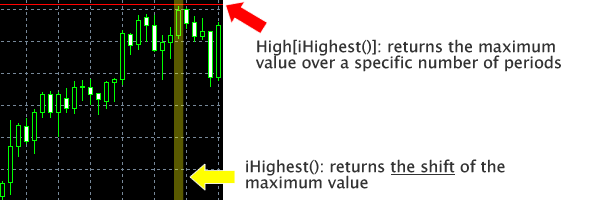## Program Contents

We will start writing the program within the following basic code.

1. int start(){
2. }

Repeating what has been said before, the command within the bracket (the curly brackets: "{ }") are the action taken each time the tick moves in the market.
Commands written inside the brackets can be broken down into three major types:

1. A command to take long position
2. A command to take short position
3. A command to settle current positions
（trailing）

## 1. Command to Take a Long Position

As stated previously, a breakout system takes a long position when the current value exceeds the maximum value of a specific time period, judging the situation as the formation of an upward trend.
Therefore, the program should take the following steps:

1. Calculate the maximum value of a set time frame
2. Compare the current value and the calculated maximum value
3. Take the long position if the current value is larger than the maximum value.

### Calculate the maximum value of a given period

Let's start with step 1: calculate the maximum value of a given period.

1. double max　=　High[iHighest(NULL, PERIOD_H1, MODE_HIGH, BreakPeriod, 1)];

The above input will yield the maximum value within a given time period set in the variable "BreakPeriod".

The function iHighest() is a function that returns the maximum value over a specific period.
For example, in the following chart, iHighest() returns the yellow band in a numerical form.
The Expert Advisor in Meta Trader, by default, prepares an array called High[]. This array contains the maximum value of each yellow bands, and the maximum value of some time period can be determined by the input High[iHighest()].The explanation may have been slightly convoluted, but it is good enough for practical purposes if you know that "the maximum value of a specific period can be determined, in data type "double", by the input High[iHighest()]